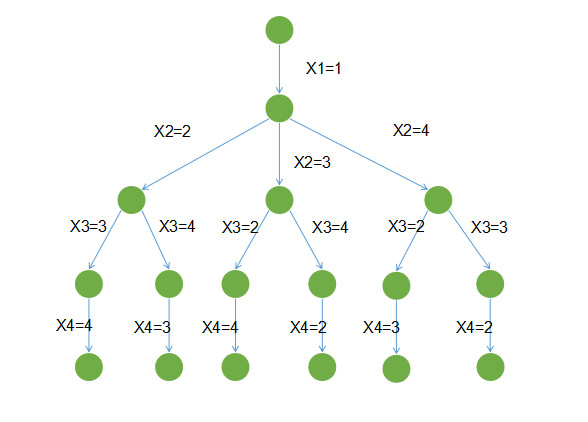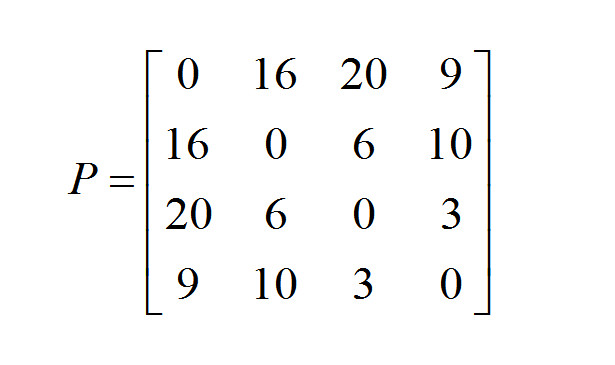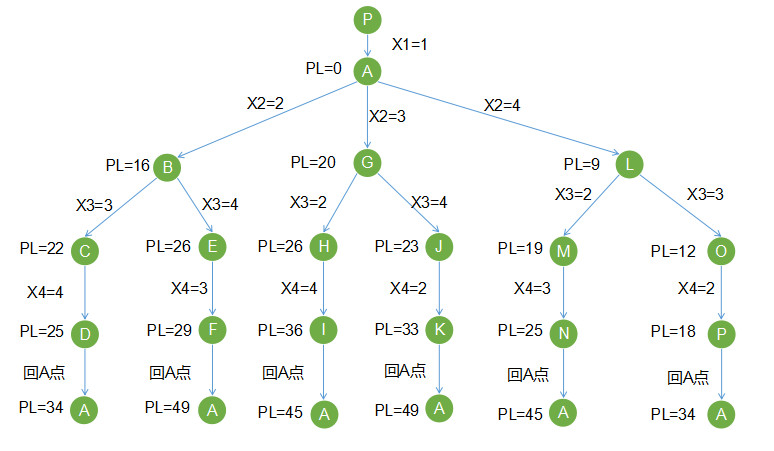# 大神告诉你如何做一名成功的AI产品经理这个职位

AI这个概念在这几年都非常火，风口之下，有许多人都想跻身这个领域，成为一名AI 产品经理。笔者通过自身经验告诉我们，想要做一名AI 产品经理，系统掌握数学和算法知识是必备步骤之一。AI（Artificial Intelligence，人工智能）这个词对大家并不陌生，甚至已经泛滥了。任何产品、任何项目、任何公司和AI沾上边似乎就会显得很高大上，可以“讲故事”。

## 一、现状

BATJ里的确有非常优秀的产品经理，但还是占少数，大多产品经理只是这些大厂众多产品的一个小螺丝钉而已，只是负责产品很小的一个点。而那些掌控全局的大厂产品经理，是非常昂贵的。

## 二、实战

### 1. 产品功能点描述

“我们看到XX外卖智能配送调度做得不错，我们现在通过人工派单效率太慢了，而且随着业务的增长，要不断新增人力，我们也需要做一个智能调度的产品，实现高效派单，提升配送效率，节省运营成本。这个事情就交给你来负责了，你就是这个智能调度产品的产品经理。”

• 输入：派送目标点数量，源送目标点间的距离
• 输出：最优路径X1=1表示第1步在编号为1的地点，X2=2，表示第2步去编号为2的地点，如果X2=3，则表示第2步去编号为3的地点。STEP1：定义矩阵P[ i ][ j ]，如果一个点 i 到 j 有边，则 P[ i ][ j ]=< i , j >，否则P[ i ][ j ]=0。

STEP2：设当前走过的路径长度为 PL，当前最优路径为BP。

STEP3：第一步从点1出发，借助辅助结点P，生成结点A，则X=1，从A点出发，则 PL=P=0。到下一派送目标点，若我们的派送目标点为2，则X=2，所以PL+P=0+16=16，PL=16 ，有效，因此生成目标点B。

STEP4：B点对应的是“图1 各派送点距离”中的点2。然后从B点出发，到下一个派送目标点3，则：PL+P=16+6=22，PL值更新为22，即PL=22，有效，因此生成目标点C。

STEP5：C点对应的是“图1 各派送点距离”中的点3。然后从C点出发，到下一个派送目标点4，则：PL+P=22+3=25，PL=25，有效，因些生成结点D。

STEP6：根据我们的前置条件，从D点直接回出发点1，则：PL+=25+9=34。这时的最优路线：BP=PL=34。找到第1条派送路线。

STEP7：之后，从生成的D点开始回溯到C点，C点满足条件的路径只有到达D点这一条，于是继续回溯至B点，B点之前是到C点（派送点3），发现B点可以至派送点4，即从B点出发，到下一个派送目标点4（结合STEP3中PL的值），则：PL+P=16+10=26，PL值更新为26，即PL=26，有效，因些生成结点E。

STEP8：从结点E（派送点4）这时满足条件的只有到派送点3，然后从派送点3回出发点1。因此：PL+P=26+3=29，PL值更新为29，即PL=29，有效，因些生成结点F。

STEP9：从F点（派送点3）回出发点1，则PL+P=29+20=49， PL更新为49。找到第2条派送路线（PL=49），但是大于之前第1条派送路线（BL=34），因此，最优路线BP仍然等于34。

STEP10：从F点（派送点3）回溯至E点（派送点4），没有满足条件的路线，继续回溯到B点（派送点2），没有满足条件的路线，继续回溯到A点（出发点1）。

STEP11：从A点（出发点1）到派送目标点3，则 PL+P=0+20=20，PL值更新为20，即PL=20，生成结点G。

STEP12：从G点到派送目标点2，则 PL+P=20+6=26，PL值更新为26，即PL=26，生成结点H。这时派送员在派送目标点2。

STEP13：从H点到派送目标点4，则PL+P=26+10=36，PL值更新为36，即PL=36，生成结点I。这时派送员在派送目标点4。

STEP13：从I点回出发点，则 PL+P=36+9=45。找到第3条派送路线（PL=45），但是大于之前第1条派送路线（BL=34），因此，最优路线BP仍然等于34。

STEP14：以同样的方法，进行回溯，回到结点G（派送点3），PL=20，派送点3之前在STEP12派送目标点是2（结点H），这次到派送目标点4，则：PL+P=20+3=23，即PL=23，生成结点J。这时派送员在派送目标点4。

STEP15：从H点到派送目标点2，则PL+P=23+10=33，PL值更新为33，即PL=33，生成结点K。这时派送员在派送目标点2。

STEP16：从K点回出发点，则 PL+P=33+16=49。找到第4条派送路线（PL=49），但是大于之前第1条派送路线（BL=34），因此，最优路线BP仍然等于34。

STEP17：从K点（派送点2）回溯至J点（派送点4），没有满足条件的路线，继续回溯到G点（派送点3），没有满足条件的路线，继续回溯到A点（出发点1）。

STEP18：继续探索新的路线。从A点（出发点1）到派送目标点4，则 PL+P=0+9=9，PL值更新为9，即PL=9，生成结点L。

STEP19：从L点到派送目标点2，则 PL+P=9+10=19，PL值更新为19，即PL=19，生成结点M。这时派送员在派送目标点2。

STEP20：从M点到派送目标点3，则 PL+P=19+6=25，PL值更新为25，即PL=25，生成结点N。这时派送员在派送目标点3。

STEP21：从M点回出发点，则 PL+P=25+20=55。找到第5条派送路线（PL=45），但是大于之前第1条派送路线（BL=34），因此，最优路线BP仍然等于34。

STEP22：以同样的方法，进行回溯，回到结点N（派送点3），再回到M点（派送点4），PL=9，派送点4之前在STEP19派送目标点是2（结点M），这次到派送目标点3，则：PL+P=9+3=12，即PL=12，生成结点O。这时派送员在派送目标点3。

STEP23：从O点到派送目标点2，则 PL+P=12+6=18，PL值更新为18，即PL=18，生成结点P。这时派送员在派送目标点2。

STEP24：从O点回出发点，则 PL+P=18+16=34。找到\第6条• QQ咨询
• 回顶